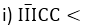#### Thank you for registering.

One of our academic counsellors will contact you within 1 working day.

Click to Chat

1800-5470-145

+91 7353221155

CART 0

• 0
MY CART (5)

Use Coupon: CART20 and get 20% off on all online Study Material

ITEM
DETAILS
MRP
DISCOUNT
FINAL PRICE
Total Price: Rs.

There are no items in this cart.
Continue Shopping

# Chapter 1: Knowing Our Numbers – Exercise 1.7

### Question: 1

Estimate the following by rounding off each factor to nearest hundreds:

i) 730 + 998

ii) 796 – 314

iii) 875 – 384

### Solution:

i) 700 + 1000 = 1700

ii) 800 – 300 = 500

iii) 900 – 400 = 500

### Question: 2

Estimate the following by rounding off each factor to nearest thousands:

i) 12904 + 2888

ii) 28292 – 21496

### Solution:

i) 13000 + 3000 = 16000

ii) 28000 – 21000 = 7000

### Question: 3

Estimate the following by rounding off each number to its greatest place:

i) 439 + 334 + 4317

ii) 8325 – 491

iii) 108734 – 47599

iv) 898 × 785

v) 9 × 795

vi) 87 × 317

### Solution:

i) 400 + 300 + 4000 = 4700

ii)8000 – 500 = 7500

iii)100000 -500000 = 50000

iv) 900 × 800 = 720000

v) 10 × 800 = 8000

vi) 90 x 300 = 27000

### Question: 4

Find the estimated quotient for each of the following by rounding off each number to its greatest place :

i) 878 ÷ 28

ii) 745 ÷ 24

iii) 4489 ÷ 394

### Solution:

i) 900 ÷ 30 = 30

ii) 700 ÷ 20 = 35

iii) 4000 ÷ 400 = 10

### Question: 5

Write the expression for each of the following statements using brackets:

i) Four multiplied by the sum of 13 and 7

ii) Eight multiplied by the difference of four from nine.

iii) Divide the difference of twenty eight and seven by 3.

The sum of 3 and 7 in multiplied by the difference of twelve and eight.

### Solution:

i) 4 × (13 + 7)

ii) 8 × (9 – 4)

iii) 28 – 73

iv) (3 + 7) x (12 – 8)

### Question: 6

Simplify each of the following:

i) 124 – (12 – 2) × 9

ii) (13 + 7) × (9 – 4) – 18

iii) 210 – (14 – 4) x (18 + 2) – 10

i) 34

ii) 82

iii) 0

### Question: 7

Simplify each of the following:

i) 7 × 109

ii) 6 × 112

iii) 9 × 105

iv) 17 × 109

v) 16 × 108

vi) 12 × 105

vii) 102 × 103

viii) 101 × 105

ix) 109 × 107

i) 763

ii) 672

iii) 945

iv) 1853

v) 1728

vi) 1260

vii) 10506

viii)10605

ix)11663

### Question: 8

Write the roman – numerals for each of the following:

i) 33

ii) 48

iii) 76

iv) 95

i) XXXIII

ii) XLVIII

iii) LXXVI

iv) XCV

### Question: 9

Write the following in roman numerals:

i) 154

ii) 173

iii) 248

iv) 319

i) CLIV

ii) CLXXIII

iii) CCXLVIII

iv) CCCXIX

### Question: 10

Write the following in roman numerals:

i) 1008

ii) 2718

iii) 3906

iv) 3794

i) KVIII

ii) KKDCCXVIII

iii) KKKCKVI

iv) KKKDCCXCIV

### Question: 11

Write the following in roman numerals:

i) 4201

ii) 10009

iii) 44000

iv) 25819

### Solution:### Question: 12

Write the following in Hindu – Arabic numerical:

i) XXVI

ii) XXIX

iii) LXXII

iv) XCI

i) 26

ii) 29

iii) 72

iv) 91

### Question: 13

Write the corresponding Hindu – Arabic numerical for each of the following:

i) CIX

ii) CLXXII

iii) CCLIV

iv) CCCXXIX

i) 109

ii) 172

iii) 254

iv) 329

### Question: 14

Write the corresponding Hindu – Arabic numerical for each of the following:

i) KXIX

ii) KDLXV

iii) KKCXXIII

iv) KKKDCXL

i) 1019

ii) 1565

iii) 2123

iv) 3640

### Question: 15

Write the following in Hindu – Arabic numerical:i) 4444

ii) 6949

iii) 9391

iv) 70009

### Question: 16

Which of the following is meaningless?ii) KKKCCXI

iii) XD

iv) VC

### Solution:

(i) and (iii) are meaningless.